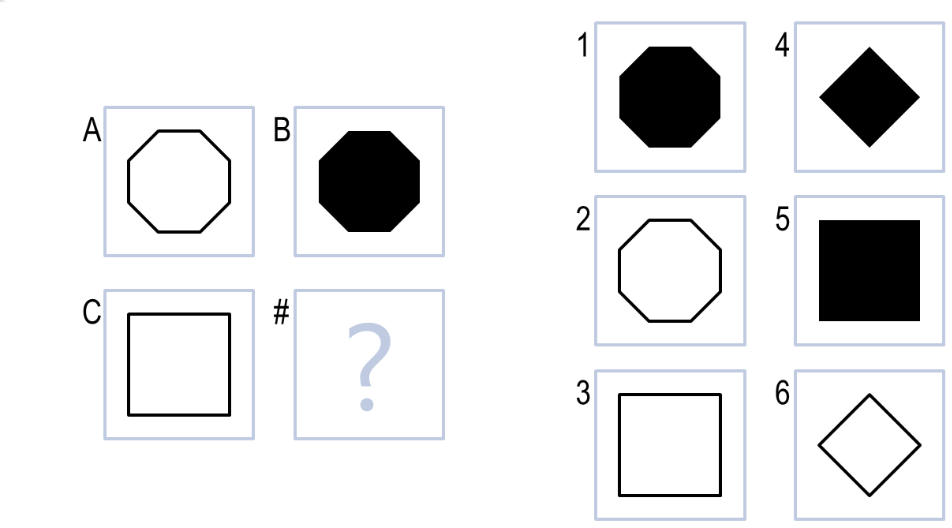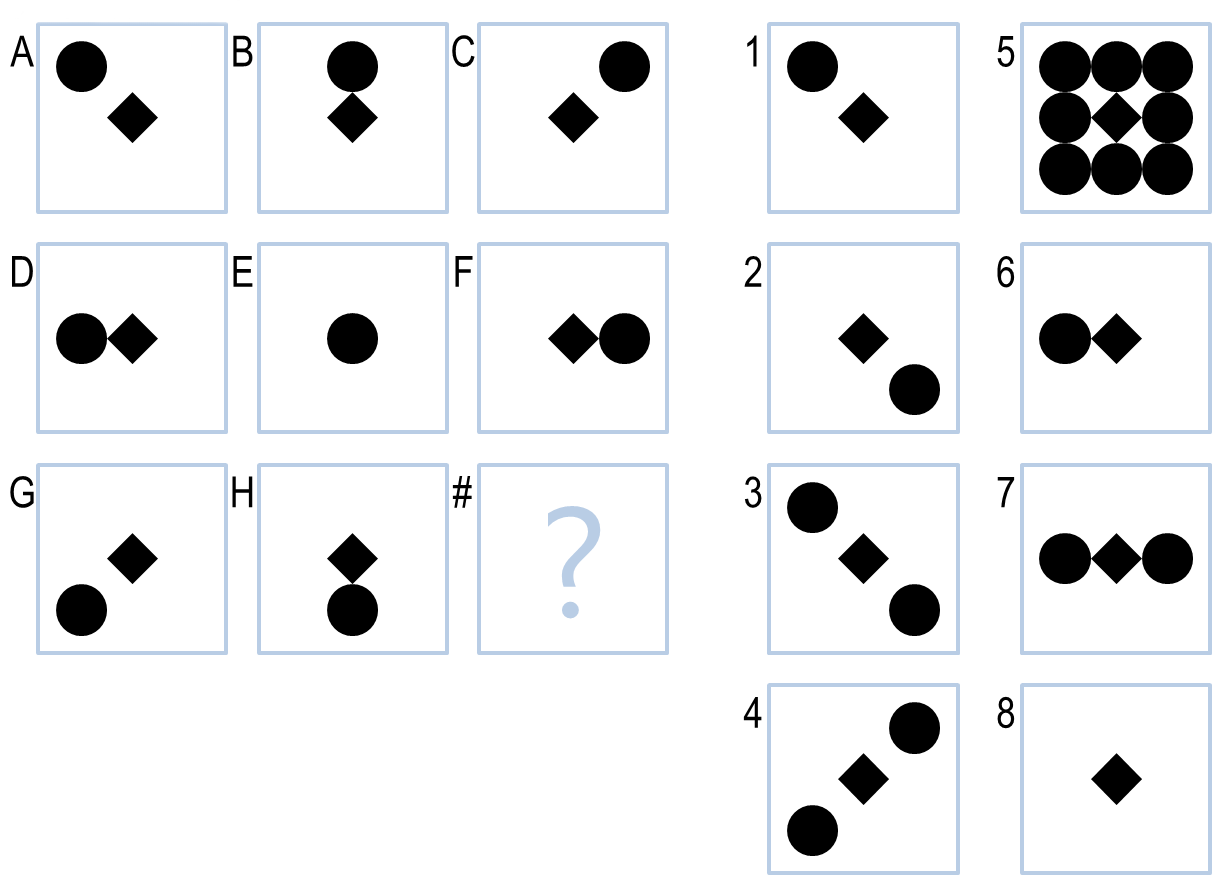# An AI Agent for Solving Raven’s Progressive Matrices

PREAMBLE Unforunate preamble here! If you are a CURRENT/FUTURE/PROSPECTIVE student at GA Tech, PLEASE DON’T EMAIL ME LOOKING FOR TIPS OR CODE IMPLEMENTATION! I won’t give you any and I’ve already had to evicerate a ton of the content in a lot of my pages because of the…interest…students have shown in the code and my approach. This blog is more a showcase and less a tutorial, especially for projects that are still being used in courses.

I took Knowledge-Based Artifical Intelligence Fall 2019 and throughly enjoyed the class projects and the ability to frontload all the course content as this class overlapped with our five week trip through Oceania. In addition to many ethics essays, the class grade was mostly based on a cummulative project using Python and Pillow to solve Raven’s Progressive Matrices. These were Professor David Joyner’s version of RPMs because of copyright issues, but the concept was the same.

### Raven’s Progressive Matices

Raven’s Progressive Matrices (RPM) are visual analogy tests used to measure abstract reasoning and fluid intelligence. Originally developed by John Raven in 1936, the test consists of 60 non-verbal pattern-matching and visual analogy questions. 2x2 or 3x3 image matrices are presented with one item missing. The task,as shown in Figure 1, is to select the visually analogous answer that best completes the matrix.Figure 1 - Raven's Matrix for a simple 2x2 problem and a more complicated 3x3 problem.

### Project 1

For Project 1, the problems were 2x2 and the transformation were often basic – a simple geometric transformation of the original image. After the images are loaded, the algorithm computes various transformations between A and B and between A and C, giving each transformation a similarity score (mean square error) between the transformed image and A.

def mse(self, a, b):
sigma_exp = .5
m,n = a.size
MSE = np.sum(np.subtract(b, a, dtype=np.float32)**2)/(m*n)
p = np.exp(-MSE / 2 / sigma_exp**2)
return (1-p)


The algorithm calculates all metrics in Table 1 for each suggested answer. It then compares these proposed matrices with the base transformation matrices Th and Tv and the best match is then chosen from the possible answers.

Table 1 - Transformations used in Project 1

Transformation Description Weight
Identity Compare A and B directly 1.00
Reflection: X Reflect B across X-axis 0.50
Reflection: Y Reflect B across Y-axis 0.50
Rotation: 90 Rotate B 90 degrees 0.25
Rotation: 180 Rotate B 180 degrees 0.25
Histogram Comparison Compare the ratio of white/black pixels 0.25

With access to only rudimentary image transformation operations (no access to OpenCV!), I tried to find easy transformations and metrics that could mimic more human-esque qualities. Humans can easily count objects in frame but it’s more difficult to separate images into distinct blobs and count the blobs. Instead of implementing blob detection, I used the ratio of white and black pixels between images. This gives a good approximation of when shapes are being added or removed while requiring almost no additional coding or computation time.

### Project 2 and 3

For project 2 and 3, the agent is asked to solve 3x3 matrices that involve more complicated transformations.

The final agent attempts to follow the same approach as the agent designed in Project 1. First, different affine transformations are calculated for horizontal and vertical figures. This includes direct transformations like A to B but also indirect, like A to C. The direct and indirect transformations are computed for D to F, E to F, E to H, and B to H. The transformation that returns the highest similarity score is chosen along with the direction that resulted in that score (direct versus indirect, horizontal or vertical). The agent then calculates the similarity between the H/F or C/G and choices 1 - 6 using only the transformation chosen in earlier steps. The option that returns the highest similarity match over a threshold value is chosen as the answer. If no answer scores over the threshold, the agent will revert to simpler weighted voting method applied in Project 1. Basic Problem C-07 (Figure 2) will be used as an example. Initially, A will be compared to B and will return the highest similarity value and the transformation that returns this score. This is repeated for the other comparison images. For C-07, Horizontal, Indirect, returns the highest score for Y-Axis Mirror across all transformations and all images. The agent would then only assess image G and the answers 1-6 for this transformation.Figure 1 - Problem solving approach using Basic Problem C-07 as an example.

### Project References

Joyner, D. B. (2015). Using Human Computation to Acquire Novel Methods for Addressing Visual Analogy Problems on Intelligence Tests. Proceedings ofthe Sixth International Conference on Computational Creativity. Provo, Utah.

### Project Reports

See the links below for all three project reports.

These were removed in 2021. Email me if you want a copy and aren’t a current KBAI student!

Tags:

Updated: